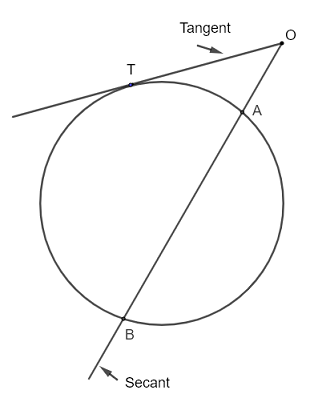# Intersecting Secant and Tangent Theorem Questions with Solutions

Consider the circle , the secants $A B$ and the tangent $OT$ in the figure below.The intersecting secant tangent theorem  states that for the secant $A B$ and tangent $O T$, there is relationship between the lengths of the segments as follows: $OT^2 = OA \times OB$

## Question With Solutions

Note that none of the figures below is drawn to scales.
Question 1
In the figure below, $OC$ is tangent to the circle. Find $x = AB$, given the lengths of segments $OC = 42$ and $OA = 21$ .Solution
Apply the intersecting secant tangent theorem above to the secant $OB$ and tangent $OC$ to write: $\quad OC^2 = OA \times OB$
Substitute the known and given quantities: $\quad 42^2 = 21 \times (21 + x)$
Expand and simplify: $\quad 1323 = 21 x$
Solve for $x$: $\quad x = 63$

Question 2
In the figure below, $OC$ is tangent to the circle. Find $a$ given the lengths of segments $OC = a - 1 , OA = a - 4, AB = a$.Solution
$OB$ is secant and $OC$ is tangent hence the intersecting secant tangent theorem gives: $\quad OC^2 = OA \times OB$
Substitute the given quantities: $\quad (a-1)^2 = (a-4)(a-4+a)$
Expand and group like terms: $\quad a^2 - 2 a + 1 = 2a^2-12a+16$
Rewrite the above quadratic equation in standard form as follows: $a^2-10a+15 = 0$
Solve for $a$ to obtain two solutions: $a=5 + \sqrt{10}$ and $a = 5 - \sqrt{10}$
Note The length of a segment must be positive. Hence $a = 5 - \sqrt{10}$ cannot be accepted as a solution because $OA = a - 4 = 5 - \sqrt{10} - 4 = 1-\sqrt{10}$ is negative
$a = 5 + \sqrt{10}$ is the only solution to the given question.

Question 3
In the figure below, $OC$ is tangent to the circle and $OE$ intersects the circle at point $D$ where $E$ is the center of the circle. The lengths of the segments $OC$ and $OD$ are given by $OD = 6$ and $OC = r + 3$ where $r$ is the length of the radius of the circle.
1) Find $r$
2) Find the lengths of the segments $OA$ and $AB$ such that $AB = 2 OA$Solution
1) Since $OC$ is tangent to the circle and $E$ is the center, then $EC$ is a radius and is perpendicular to $OC$.Use the Pythagorean theorem to write: $\quad (OD + DE)^2 = EC^2 + OC^2$
Note that $DE$ is a radius and substitute by the given quantities: $\quad (6+r)^2 = r^2 + (r+3)^2$
Expand and write the above equation in standard form: $\quad -r^2+6r+27 = 0$
Solve for $r$ to obtain two solutions: $r = -3$ and $r = 9$ and only the solution $r = 9$ is valid since the length of the radius is a positive quantity.
2) The use of the intersecting secant tangent theorem gives: $\quad OC^2 = OA \times OB$
Substitute the known and unknown quantities: $\quad (9 + 3)^2 = OA \times (O A + 2 O A)$
The above may be written as $\quad (15)^2 = 3 OA^2$
Solve for $OA$: $\quad OA = \dfrac{15}{\sqrt 3}$ , $AB = 2 \dfrac{15}{\sqrt 3}$